# Texas Go Math Grade 5 Lesson 13.5 Answer Key Multi-Step Measurement Problems

Refer to our Texas Go Math Grade 5 Answer Key Pdf to score good marks in the exams. Test yourself by practicing the problems from Texas Go Math Grade 5 Lesson 13.5 Answer Key Multi-Step Measurement Problems.

## Texas Go Math Grade 5 Lesson 13.5 Answer Key Multi-Step Measurement Problems

Essential Question
How can you solve multi-step problems that include measurement conversions?
The steps to solve the multi-step problems that include measurement conversions are:
Step 1:
Write the given information and what to find
Step 2:
Plan a strategy how to find the required information or quantity
Step 3:
If there are unit conversions, then convert them by using the following rules:
If we have to convert a small unit into a larger unit, then multiply
If we have to convert a larger unit into a small unit, then divide

Unlock the Problem

A leaky faucet in Jarod’s house drips 2 cups of water each day. After 2 weeks of dripping, the faucet is fixed. ¡fit dripped the same amount each day, how many quarts of water dripped from Jarod’s leaky faucet in 2 weeks?

Use the steps to solve the multi-step problem.
Step 1
Record the information you are given.
The faucet drips 2 cups of water each day.
The faucet drips for 2 weeks.

Step 2
Find the total amount of water dripped in cups each day days in 2 weeks.
Since you are given the amount of water dripped each day, you must convert 2 weeks into days and multiply.
Think: There are 7 days in 1 week.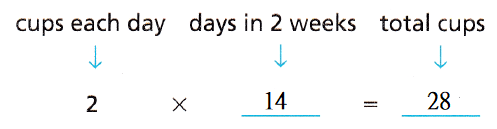So,
The faucet drips 14 cups in 2 weeks.

Step 3
Convert from cups to quarts.
Think: There are 2 cups in 1 pint.
There are 2 pints in 1 quart.
2 cups = 2 pints
2 pints = 4 quarts
So,
Jarod’s leaky faucet drips 56 quarts of water in 2 weeks.

• What if the faucet dripped for 4 weeks before it was fixed? How many quarts of water would have leaked?
From the above Problem,
We can observe that
Jarod’s leaky faucet drips 56 quarts of water in 2 weeks.
So,
The number of quarts of water if the faucet dripped for 4 weeks = 2 × (The number of quarts of water if the faucet dripped for 2 weeks)
= 2 × 56
= 112 quarts
Hence, from the above,
We can conclude that
The number of quarts of water if the faucet dripped for 4 weeks is: 112 quarts of water

Example
A carton of large, Grade A eggs weighs about 1.5 pounds. If a carton holds a dozen eggs, how many ounces does each egg weigh?Step 1
In ounces, find the weight of a carton of eggs.
Think: 1 pound = 16 ounces
Weight of a carton (in ounces):So,
The carton of eggs weighs about 24 ounces.
Step 2
In ounces, find the weight of each egg in a carton.
Think: 1 carton (dozen eggs) = 12 eggs
Weight of each egg (in ounces):So,
Each egg weighs about 2 ounces.

Share and Show

Question 1.
After each soccer practice, Scott runs 4 sprints of 20 yards each. If he continues his routine, how many practices will it take for Scott to have sprinted a total of 2 miles combined?
Scott sprints ___ yards each practice.
Since there are ___ yards in 2 miles, he will need to continue his routine for ___ practices.
It is given that
After each soccer practice, Scott runs 4 sprints of 20 yards each.
So,
The number of yards Scott sprints each practice = 4 × 20
= 80 yards
Now,
We know that,
1 mile = 1,760 yards
So,
The number of practices will it take for Scott to have sprinted a total of 2 miles = $$\frac{2 × 1,760}{80}$$
= 44 practices
Hence, from the above,
We can conclude that
The number of practices will it take for Scott to have sprinted a total of 2 miles is: 44 practices

A worker at a mill is loading 5-lb bags of flour into boxes to deliver to a local warehouse. Each box holds 12 bags of flour. If the warehouse orders 3 tons of flour, how many boxes are needed to fulfill the order?
It is given that
A worker at a mill is loading 5-lb bags of flour into boxes to deliver to a local warehouse. Each box holds 12 bags of flour
Now,
According to the given information,
The total number of bags of flour = (The total number of bags) × 5
= 5 × 12
= 60 bags of flour
Now,
We know that,
1 Ton = 2,000 Pounds
So,
The total number of boxes needed to fulfill the given order = (The total quantity of the order) ÷ (The number of bags of flour)
= $$\frac{3 × 2,000}{60}$$
= $$\frac{6,000}{60}$$
= 100 boxes
Hence, from the above,
We can conclude that
The total number of boxes needed to fulfill the given order is: 100 boxes

Question 3.
Cory brings five 1-gallon jugs of juice to serve during parent night at his school. If the paper cups he is using for drinks can hold 8 fluid ounces, how many drinks can Cory serve for parent night?
It is given that
Cory brings five 1-gallon jugs of juice to serve during parent night at his school
Now,
We know that,
1 Gallon = 4 Quarts
1 Quart = 2 Pints
1 Pint = 2 cups
1 cup = 8 fluid ounces
So,
1 Gallon = 4 × 2 × 2 × 8
= 8 × 16
= 128 fluid ounces
Now,
The number of drinks that Cory can serve for Parent night = $$\frac{128}{8}$$
= 16 drinks
Hence, from the above,
We can conclude that
The number of drinks that Cory can serve for Parent night is: 16 drinks

Math Talk
Mathematical Processes
Explain the steps you took to solve Exercise 2?
The steps that you took to solve Exercise 2 are:
Step 1:
Find the number of bags of flour
Step 2:
Convert 3 tons into pounds
Step 3:
Divide the result in Step 3 and the result in Step 2 respectively to find the number of boxes that are needed to fulfill the order

Problem Solving

Solve.

Question 4.
Apply A science teacher needs to collect lake water for a lab she is teaching about purifying water. The lab requires each student to use 4 fluid ounces of lake water. If 68 students are participating, how many pints of lake water will the teacher need to collect?
It is given that
A science teacher needs to collect lake water for a lab she is teaching about purifying water. The lab requires each student to use 4 fluid ounces of lake water
Now,
We know that,
1 cup = 8 fluid ounces
1 pint = 2 cups
So,
4 fluid ounces = $$\frac{1}{4}$$ pint
So,
According to the given information,
The number of pints of lake water the teacher will be needed to collect  = 68 × 4 fluid ounce
= 272 fluid ounces
= $$\frac{272}{16}$$
= 17 pints
Hence, from the above,
We can conclude that
The number of pints of lake water the teacher will need to collect is: 17 pints

H.O.T. Use Diagrams A string of decorative lights is 28 feet long. The first light on the string is 16 inches from the plug. If the lights on the string are spaced 4 inches apart, how many lights are there on the string? Draw a picture to help you solve the problem.
It is given that
A string of decorative lights is 28 feet long. The first light on the string is 16 inches from the plug and the lights on the string are spaced 4 inches apart
Now,
We know that,
1 feet = 12 inches
So,
The total length of a string = 28 × 12
= 336 inches
Now,
The remaining length of the string = (The total length of the string) – (The distance of the first light on the string)
= 336 – 16
= 320 inches
So,
The total number of lights that are present on the string = (The remaining length of the string) ÷ (The distance of each light placed)
= $$\frac{320}{4}$$
= 80 lights
Hence, from the above,
We can conclude that
The total number of lights that are present on the string is: 80 lights

Question 6.
Multi-Step When Jamie’s car moves forward such that each tire makes one full rotation, the car has traveled 72 inches. How many full rotations do the tires make when Jamie’s car travels 10 yards?
It is given that
When Jamie’s car moves forward such that each tire makes one full rotation, the car has traveled 72 inches
Now,
The distance travelled in one rotation = 72 inches,
So,
The number of rotation the car travelled for 72 inches = 1
Now,
The number of rotations the car travelled for 1 inches= $$\frac{1}{72}$$
Also,
It is given that
The car travelled 10 yards
Now,
We know that,
1 yard = 36 inches
So,
The distance travelled by the car = 36 × 10
= 360 inches
So,
The number of rotations the car travelled for 360 inches = $$\frac{360}{72}$$
= 5 rotations
Hence, from the above,
We can conclude that
The number of rotations the car travelled for 360 inches is: 5 rotations

Question 7.
Multi-Step A male African elephant weighs 7 tons. If a male African lion at the local zoo weighs 13,650 pounds less than the male African elephant, how many pounds does the lion weigh?
It is given that
A male African elephant weighs 7 tons and a male African lion at the local zoo weighs 13,650 pounds less than the male African elephant
Now,
We know that,
1 Ton = 2,000 pounds
So,
The weight of a male African elephant = 7 × 2,000 pounds
= 14,000 pounds
Now,
According to the given information,
The weight of a male African lion = 14,000 – 13,650
= 350 Pounds
Hence, from the above,
We can conclude that
The weight of a male African elephant is: 350 pounds

Problem Solving

Question 8.
Multi-Step An office supply company is shipping a case of pencils to a store. There are 64 boxes of pencils in the case. If each box of pencils weighs 2.5 ounces, what is the weight, in pounds, of the case of pencils?
It is given that
An office supply company is shipping a case of pencils to a store. There are 64 boxes of pencils in the case. If each box of pencils weighs 2.5 ounces
So,
The total weight of the case of pencils = 64 × (The weight of each box of pencils)
= 64 × 2.5
= 160 ounces
Now,
We know that,
1 pound = 16 ounces
1 ounce = $$\frac{1}{16}$$ pounds
So,
The total weight of the case of pencils in pounds = 160 × $$\frac{1}{16}$$
= 10 pounds
Hence, from the above,
We can conclude that
The total weight of the case of pencils in pounds is: 10 pounds

H.O.T. At a local animal shelter, there are 12 small-size dogs and 5 medium-size dogs. Every day, the small-size dogs are each given 12.5 ounces of dry food and the medium-size dogs are each given 18 ounces of the same dry food. How many pounds of dry food does the shelter serve in one day?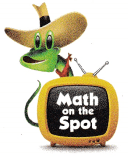It is given that
At a local animal shelter there are 12 small-size dogs and 5 medium-size dogs. Every day, the small-size dogs are each given 12.5 ounces of dry food and the medium-size dogs are each given 18 ounces of the same dry food.
Now,
According to the given information,
The number of ounces of dry food does the shelter serve in one day = (12 × 12.5) + (5 × 18)
= 150 + 90
= 240 ounces
Now,
We know that,
1 Pound = 16 ounces
1 ounce = $$\frac{1}{16}$$ pound
So,
The number of pounds of dry food does the shelter serve in one day = 240 × $$\frac{1}{16}$$ pounds
= 15 pounds
Hence, from the above,
We can conclude that
The number of pounds of dry food does the shelter serve in one day is: 15 pounds

Question 10.
Analyze A 2.3-ton shark is on one side of a pan balance in a cartoon. Seals are piling up on the other side, trying to “outweigh” the shark. What is the least number of 150-pound seals that would have a combined weight greater than the shark’s weight?
(A) 28 seals
(B) 30 seals
(C) 29 seals
(D) 31 seals
It is given that
A 2.3-ton shark is on one side of a pan balance in a cartoon. Seals are piling up on the other side, trying to “outweigh” the shark
Now,
We know that,
1 Ton = 2,000 Pounds
So,
The weight of a shark = 2.3 × 2,000 pounds
= 4,600 pounds
So,
According to the given information,
The least number of 150-pound seals that would have a combined weight greater than the shark’s weight = $$\frac{4,600}{150}$$
= 30.66
≅ 31 seals
Hence, from the above,
We can conclude that
The least number of 150-pound seals that would have a combined weight greater than the shark’s weight is: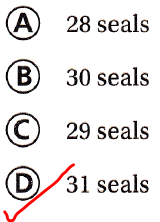Question 11.
Sara has 2 pounds of chicken. She uses 5 ounces of chicken while cooking. How can you determine the mass of chicken that she has left?
(A) Divide 2 by 16, and then subtract 5.
(B) Divide 2 by 16, and then add 5.
(C) Multiply 2 by 16, and then subtract 5.
(D) Multiply 2 by 16, and then add 5.
It is given that
Sara has 2 pounds of chicken. She uses 5 ounces of chicken while cooking
Now,
We know that,
1 pound = 16 ounces
So,
The total amount of chicken Sara has = 2 × 16
= 32 ounces
Now,
The mass of the chicken that Sara has left = (16 × 2) – 5
= 32 – 5
= 27 ounces
Hence, from the above,
We can conclude that
The mass of the chicken that Sara has left is:Multi-Step Leo has three pieces of ribbon. One piece is 4 yards long, another piece is 5 feet long, and the last piece is 10 inches long. How much ribbon does Leo have in all?
(A) 214 inches
(B) 118 inches
(C) 19 inches
(D) 57 inches
It is given that
Leo has three pieces of ribbon. One piece is 4 yards long, another piece is 5 feet long, and the last piece is 10 inches long
Now,
We know that,
1 yard = 36 inches
1 feet = 12 inches
So,
The total length of the ribbon = (4 × 36) + (5 × 12) + 10
= 144 + 60 + 10
= 214 inches
Hence, from the above,
We can conclude that
The total length of the ribbon is: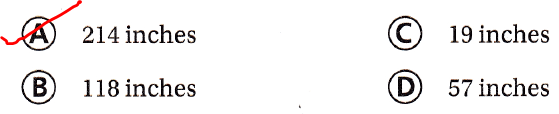Texas Test Prep

Question 13.
For a class assignment, students are asked to record the total amount of water they drink in one day. Melinda records that she drank four 8-fluid ounce glasses of water and two 1-pint bottles. How many quarts of water did Melinda drink during the day?
(A) 2 quarts
(B) 4 quarts
(C) 3 quarts
(D) 8 quarts
It is given that
For a class assignment, students are asked to record the total amount of water they drink in one day. Melinda records that she drank four 8-fluid ounce glasses of water and two 1-pint bottles
Now,
We know that,
1 pint = 16 fluid ounces
1 quart = 32 fluid ounces
1 fluid ounce = $$\frac{1}{32}$$ quarts
Now,
According to the given information,
The number of quarts of water did Melinda drink during the day = (4 × 8 fluid ounces) + (2 × 1 pint)
= (32 × $$\frac{1}{32}$$ quarts) + (2 × $$\frac{1}{2}$$ quarts)
= 1 + 1
= 2 quarts
Hence, from the above,
We can conclude that
The number of quarts of water did Melinda drink during the day is: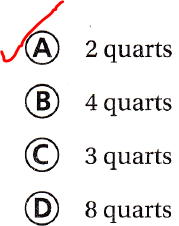### Texas Go Math Grade 5 Lesson 13.5 Homework and Practice Answer Key

Solve.

Question 1.
Diego drinks 16 ounces of his favorite sport drink every day after soccer practice for 2 weeks. How many quarts of the sport drink does Diego consume in 2 weeks?
It is given that
Diego drinks 16 ounces of his favorite sport drink every day after soccer practice for 2 weeks
Now,
We know that,
1 cup = 8 fluid ounces
1 pint = 2 cups
1 quart = 2 pints
1 week = 7 days
1 quart = 32 ounces
So,
The number of quarts of the sport drink does Diego consume in 2 weeks = 2 × 7 ×  $$\frac{16}{32}$$
= 14 × $$\frac{1}{2}$$
= 7 quarts
Hence, from the above,
We can conclude that
The number of quarts of the sport drink does Diego consume in 2 weeks is: 7 quarts

Question 2.
A rancher is putting a fence around a square animal pen. The perimeter of the pen is 32 feet. The fence posts will be 16 inches apart. She starts by putting 1 fence post at each corner of the pen. How many fence posts does she use altogether? Draw a picture to model the problem.
It is given that
A rancher is putting a fence around a square animal pen. The perimeter of the pen is 32 feet. The fence posts will be 16 inches apart. She starts by putting 1 fence post at each corner of the pen
Now,
We know that,
1 feet = 12 inches
So,
The perimeter of the pen = 32 × 12
= 384 inches
So,
The number of fence posts does a rancher used altogether = $$\frac{384}{16}$$
= 24 fence posts
Hence, from the above,
We can conclude that
The number of fence posts a rancher uses altogether is: 24 fence posts

A koala weighs 20 pounds. In her pouch, she carries her joey which weighs 20 ounces. What is the combined weight of the adult koala and her joey in ounces?
It is given that
A koala weighs 20 pounds. In her pouch, she carries her Joey which weighs 20 ounces
Now,
We know that,
1 pound = 16 ounces
So,
The combined weight of the adult koala and her joey in ounces = (20 × 16) + 20
= 320 + 20
= 340 ounces
Hence, from the above,
We can conclude that
The combined weight of the adult koala and her joey in ounces is: 340 ounces

Question 4.
On Friday, 32 students in Mr. Tanika’s class are each served 6 ounces of milk for lunch. How many quarts of milk are served to the class on Friday?
It is given that
On Friday, 32 students in Mr. Tanika’s class are each served 6 ounces of milk for lunch
Now,
We know that,
1 quart = 32 ounces
So,
The number of quarts of milk are served to the class on Friday = 32 × 6 ounces
= 192 ounces
= $$\frac{192}{32}$$
= 6 quarts
Hence, from the above,
We can conclude that
The number of quarts of milk are served to the class on Friday is: 6 quarts

Problem Solving

Question 5.
Vanessa bought 5 feet of ribbon. She cut off 36 inches to wrap a package and 18 inches to decorate her scrapbook. How much ribbon does Vanessa have left?
It is given that
Vanessa bought 5 feet of ribbon. She cut off 36 inches to wrap a package and 18 inches to decorate her scrapbook
Now,
We know that,
1 feet = 12 inches
So,
According to the given information,
The amount of ribbon does Vanessa has left = (5 × 12) – (36 + 18)
= 60 – 54
= 6 inches
Hence, from the above,
We can conclude that
The amount of ribbon does Vanessa has left is: 6 inches

Question 6.
Students fill beanbags to play a classroom number game. Each beanbag contains 3 cups of beans. They have a 1-pint container, a 1-quart container, and a 1-gallon container filled with beans to use for the beanbags. What is the greatest number of beanbags they can make?
It is given that
Students fill beanbags to play a classroom number game. Each beanbag contains 3 cups of beans. They have a 1-pint container, a 1-quart container, and a 1-gallon container filled with beans to use for the beanbags
Now,
We know that,
1 pint = 2 cups
1 quart = 2 pints
1 gallon = 4 quarts
So,
The number of beanbags present in a 1-pint container = 3 × 2 = 6 beanbags
The number of beanbags present in a 1-quart container = 3 × 2 × 6 = 36 beanbags
The number of beanbags present in a 1-gallon container = 3 × 4 × 36 = 432 beanbags
Hence, from the above,
We can conclude that
The greatest number of beanbags the students can make is: 432 beanbags

Texas Prep

Lesson Check

Question 7.
A large brick weighs 8 pounds. A small brick weighs 52 ounces. How can you determine the difference in weight between the large brick and the small brick?
(A) Divide 16 by 8, and then add 52.
(B) Divide 16 by 8, and then subtract 52.
(C) Multiply 8 by 16, and then add 52.
(D) Multiply 8 by 16, and then subtract 52.
It is gievn that
A large brick weighs 8 pounds. A small brick weighs 52 ounces
Now,
We know that,
1 Pound = 16 Ounces
So,
According to the given information,
The difference in weight between the large brick and the small brick = (8 × 16) – 52
Hence, from the above,
We can conclude that
The difference in weight between the large brick and the small brick is given by:Go Math Multi Step Word Problems 5th Grade Question 8.
Ariel has a gallon container of juice and a one-quart container of juice. How many 1-cup servings of juice does Ariel have?
(A) 20
(B) 36
(C) 12
(D) 44
It is given that
Ariel has one-gallon container of juice and one-quart container of juice
Now,
We know that,
1 pint = 2 cups
1 quart = 2 pints
1 gallon = 4 quarts
So,
According to the given information,
The number of 1-cup servings of juice does Ariel have = (1 × 4 × 2) + (1 × 4)
= 8 + 4
= 12 1-cup servings
Hence, from the above,
We can conclude that
The number of 1-cup servings of juice does Ariel have is:Question 9.
The veterinarian weighs Jim’s two dogs. His collie weighs 37 pounds. His terrier weighs 240 ounces. What is the total weight of Jim’s dogs?
(A) 592 ounces
(B) 52 pounds
(C) 53 pounds
(D) 12 pounds
It is given that
The veterinarian weighs Jim’s two dogs. His collie weighs 37 pounds. His terrier weighs 240 ounces
Now,
We know that,
1 pound = 16 ounces
So,
The total weight of Jim’s dogs = (37 × 16)+ 240
= 592 + 240
= 832 ounces
= $$\frac{832}{16}$$
= 52 pounds
Hence, from the above,
We can conclude that
The total weight of Jim’s dogs is:Question 10.
An elevator has a maximum weight limit of 1.5 tons. Rozell Company workers load boxes that weigh 150 pounds each into the elevator to transport them to the first floor. What is the greatest number of boxes the elevator can hold?
(A) 16
(B) 13
(C) 10
(D) 20
It is given that
An elevator has a maximum weight limit of 1.5 tons. Rozell Company workers load boxes that weigh 150 pounds each into the elevator to transport them to the first floor
Now,
We know that,
1 Ton = 2,000 Pounds
So,
According to the given information,
The greatest number of boxes the elevator can hold = $$\frac{1.5 × 2,000}{150}$$
= 20 boxes
Hence, from the above,
We can conclude that
The greatest number of boxes the elevator can hold is: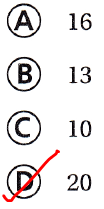Question 11.
Multi-Step An artist painted a rectangular mural on the wall. Two sides are each 26 inches long. The other two sides are each 2 yards long. What is the perimeter of the mural?
(A) 98 inches
(B) 196 inches
(C) 62 inches
(D) 144 inches
It is given that
An artist painted a rectangular mural on the wall. Two sides are each 26 inches long. The other two sides are each 2 yards long
Now,
We know that,
1 feet = 12 inches
1 yard = 36 inches
So,
According to the given information,
The perimeter of the mural = (2 × 26) + (2 × 2 × 36)
= 52 + 144
= 196 inches
Hence, from the above,
We can conclude that
The perimeter of the mural is:Question 12.
Multi-Step Vince has a rectangular rug in his room with an area of 10 feet. The length of the rug is 18 inches longer than the width. What could be the dimensions of the rug?
(A) length: 4 feet; width 2.5 feet
(B) length: 2 feet; width 3 feet
(C) length: 5 feet; width 2 feet
(D) length: 2.5; width 4 feet
It is given that
Vince has a rectangular rug in his room with an area of 10 feet. The length of the rug is 18 inches longer than the width
Now,
We know that,
1 feet = 12 inches
Now,
Let the width of the rectangular rug be x inches
So,
According to the given information,
10 = x (x + 1.5)
Hence, from the above,
We can conclude that
The dimensions of the rug are:Scroll to Top